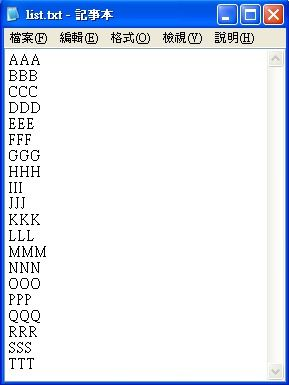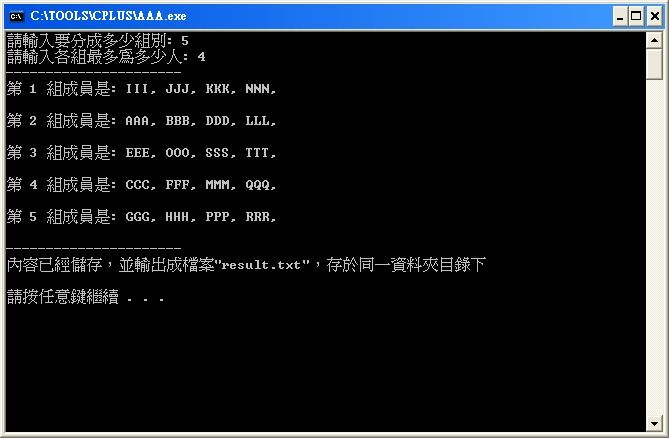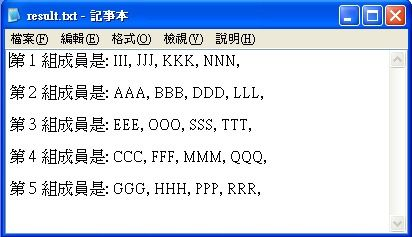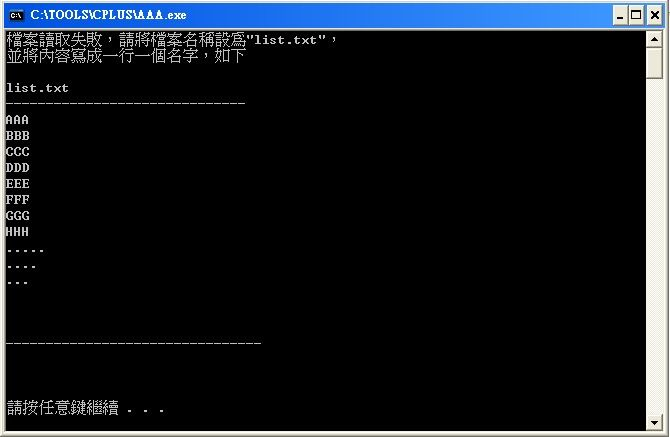-------------------------------------------------------------------------------

txt給程式 -->  程式執行 --> 輸出txt

```#include <cstdlib>
#include <fstream>
#include <iostream>
#include <ctime>
#include <cstring>```

```#include <cstdlib>
#include <fstream>
#include <iostream>
#include <ctime>
#include <cstring>

using namespace std;

int main()
{
system("pause");
return 0;
}
```

using namespace std;，代表的是名稱空間，這一行的功用即是在告訴編譯器，所需要的類別或功能要去這個名稱空間(std)就能找到，一般預設都是std ，所以照抄即可，若少了這一可是會在編譯時冒出一堆錯誤呢@@

```fstream file1;
file1.open("list.txt");```

```int team_count = 0;
int team_member_count = 0;
cout << "請輸入要分成多少組別: " ;
cin >> team_count;
cout << "請輸入各組最多為多少人: ";
cin >> team_member_count;
```

```string first = "第一組成員為：";
string second = "第二組成員為：";
string third = "第三組成員為：";
```

不過這樣子完全沒效率，也非動態產生，也看不出剛剛輸入的組別數有被確實的使用到，所以改寫成如下

```string ss[team_count];
```

```int *ccc = NULL;
ccc = new int[team_count];

......
delete [] ccc; //歸還記憶體
```

```    for(int m = 0; m<team_count; m++){
char mm;
itoa( m+1, mm, 10 ); //int 轉成 char
ss[m] = ss[m] + "第 " + mm + " 組成員是: ";      //給予陣列起始的內容
}
```

```     int *count = NULL;
count = new int[team_count];
for(int i = 0; i<team_count;i++){
count[i] = 0;  //數字陣列內容先全部給0
}
```

```    #define size 70
char oneline[size];
bool flag = true;
srand(time(NULL));

while(file1.getline(oneline,sizeof(oneline), '\n')){
flag = true;
while(flag){
int i =  (rand() % team_count) ;
if(count[i] != team_member_count){
count[i]++;
ss[i] = ss[i] + oneline +", ";
flag = false;
}
}
}
````file1.getline(oneline,sizeof(oneline), '\n')`

```    ofstream file2;
file2.open("result.txt");
cout << "----------------------\n";
for(int j=0; j<team_count; j++){
cout << ss[j] << "\n\n" ;
file2 << ss[j] << "\n\n" ;
}
file1.close();
file2.close();
cout << "----------------------\n";
cout <<"內容已經儲存，並輸出成檔案\"result.txt\"，存於同一資料夾目錄下\n\n";
```

```    file1.close();
file2.close();
```

```#include <cstdlib>
#include <fstream>
#include <iostream>
#include <ctime>
#include <cstring>

using namespace std;

int main()
{
//先建立檔案資料流，並讀取檔案
fstream file1;
file1.open("list.txt");
//判斷檔案是不是有被讀到
if(file1 != NULL){
// 從使用者的輸入來取得要分成幾組及各組要多少人
int team_count = 0;
int team_member_count = 0;
cout << "請輸入要分成多少組別: " ;
cin >> team_count;
cout << "請輸入各組最多為多少人: ";
cin >> team_member_count;

string ss[team_count];  //建立字串陣列，大小為組別數
for(int m = 0; m<team_count; m++){
char mm;
itoa( m+1, mm, 10 ); //int 轉成 char
ss[m] = ss[m] + "第 " + mm + " 組成員是: ";      //給予陣列起始的內容
}

//借用記憶體，並建立動態的數字陣列
int *count = NULL;
count = new int[team_count];
for(int i = 0; i<team_count;i++){
count[i] = 0;  //數字陣列內容先全部給0
}

#define size 100
char oneline[size];
bool flag = true;
srand(time(NULL));

//開始一行一行讀取檔案
while(file1.getline(oneline,sizeof(oneline), '\n')){
flag = true;
while(flag){
int i =  (rand() % team_count) ;
if(count[i] != team_member_count){
count[i]++;
ss[i] = ss[i] + oneline +", ";
flag = false;
}
}
}

//將分組結果輸出 ，同時列印出結果
ofstream file2;
file2.open("result.txt");
cout << "----------------------\n";
for(int j=0; j<team_count; j++){
cout << ss[j] << "\n\n" ;
file2 << ss[j] << "\n\n" ;
}
file1.close();
file2.close();
cout << "----------------------\n";
cout <<"內容已經儲存，並輸出成檔案\"result.txt\"，存於同一資料夾目錄下\n\n";
}else{
cout <<"檔案讀取失敗，請將檔案名稱設為\"list.txt\"，\n並將內容寫成一行一個名字，如下\n\n";
cout <<"list.txt\n------------------------------\n";
cout <<"AAA\nBBB\nCCC\nDDD\nEEE\nFFF\nGGG\nHHH\n.....\n....\n...\n\n\n\n--------------------------------\n\n\n\n";
}

system("pause");
return 0;
}
//程式到此結束```